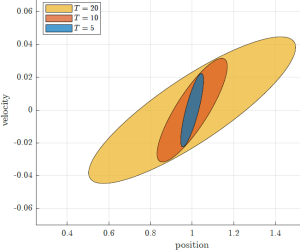ME 7247
Northeastern University

Instructor: Laurent LessardThis is a graduate-level course that covers topics in modern control engineering, including: optimal control, optimal filtering, robust/nonlinear control, and model predictive control. The main theme of the course is how uncertainty propagates through dynamical systems, and how it can be managed in the context of a control system. We will emphasize modern tools from computational linear algebra and convex optimization, and use Matlab for implementation. List of prerequisites:

• Required: Basic linear algebra / differential equations; matrix/vector manipulations, subspaces, linear independence, solving ODEs and systems of linear equations. (e.g., MATH 2341)
• Recommended: Basic linear systems theory; state-space equations, controllability/observability. (e.g. ME 5659 or EECE 7200).
• Strongly recommended: Experience using Matlab. If you know how to use other scripting languages such as Python+Matplotlib, you’ll find Matlab very easy to learn and you should be fine.
No textbook or software purchase required; everything you need will be provided.

IMPORTANT: The notes below are from Fall 2022-23, which was the last time Prof. Lessard taught this course. These are scribed notes; they were written by students enrolled in the class and subsequently edited by Prof. Lessard. As the course evolves, the notes will continue to improve!